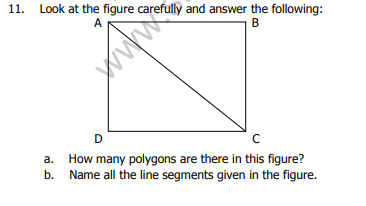# CBSE Class 4 Mathematics Sample Paper Set D

Read and download PDF of CBSE Class 4 Mathematics Sample Paper Set D designed as per the latest curriculum and examination pattern for Class 4 issued by CBSE, NCERT and KVS. The latest Class 4 Mathematics Sample Papers have been provided with solutions so that the students can solve these practice papers and then compare their answers. This will help them to identify mistakes and improvement areas in Mathematics Standard 4 which they need to study more to get better marks in Grade 4 exams. After solving these guess papers also refer to solved Class 4 Mathematics Question Papers available on our website to build strong understanding of the subject

## Sample Paper for Class 4 Mathematics Pdf

Students can refer to the below Class 4 Mathematics Sample Paper designed to help students understand the pattern of questions that will be asked in Grade 4 exams. Please download CBSE Class 4 Mathematics Sample Paper Set D

### Mathematics Class 4 Sample Paper

CBSE Class 4 Mathematics Sample Paper Set D. It’s always recommended to practice as many CBSE sample papers as possible before the examinations. The latest sample papers have been designed as per the latest blue prints, syllabus and examination trends. Sample papers should be practiced in examination condition at home or school and also show it to your teachers for checking or compare with the answers provided. Students can download the sample papers in pdf format free and score better marks in examinations. Refer to other links too for latest sample papers.

1. Mental Maths :

a. The largest factor of a number is the number _____________

b. The digit in the ones place should be _______ or _______ for the number to bedivisible by 5

c. Multiples of an even number are all ______________ numbers.

d. Circle the factors of 35 – 1, 2, 5, 7, 30, 35

e. A line segment has __________ end points.

f. 100 is a _____________ of 5.( Factor/ Multiple)

2. List all the prime numbers from 70 – 80

3. Is 2495 divisible by 10? Give reason.

4. Make a factor tree of 63.

5. Draw and name a line segment of 7.5cm

6. Check if 8 is a factor of 42?

7. Give reason whether 549036 is an even or an odd number?

8. Using multiplication find the factors of 84

9. Write the first 6 multiples of 13.

10. Draw the following: